## Fontené Theorems

1. If the sides of the Pedal Triangle of a point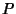meet the corresponding sides of a Triangleat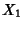,, and, respectively, then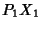,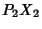,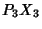meet at a pointcommon to the Circles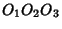and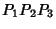. In other words,is one of the intersections of the Nine-Point Circle ofand the Pedal Circle of.

2. If a point moves on a fixed line through the Circumcenter, then its Pedal Circle passes through a fixed point on the Nine-Point Circle.

3. The Pedal Circle of a point is tangent to the Nine-Point Circle Iff the point and its Isogonal Conjugate lie on a Line through the Orthocenter. Feuerbach's Theorem is a special case of this theorem.

See also Circumcenter, Feuerbach's Theorem, Isogonal Conjugate, Nine-Point Circle, Orthocenter, Pedal Circle

References

Johnson, R. A. Modern Geometry: An Elementary Treatise on the Geometry of the Triangle and the Circle. Boston, MA: Houghton Mifflin, pp. 245-247, 1929.